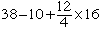Hello My Name is Brenda. i am neither a student or a teacher, but a mother who's math skills are rusty. my math question is as follows: 38-10+12divided by4multiplied by 16=? Thank-you Brenda Hi Brenda, I think the expression you describe isWhen evaluating mathematical expressions the convention is to perform divisions, then multiplications followed by additions and subtractions. Thus, since  12/4 = 3, evaluating your expression I get 38 - 10 + 3 x 16 = 38 - 10 + 48 = 28 + 48 =76 Penny Go to Math Central